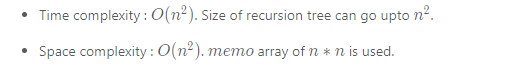#0

## Longest Increasing Subsequence (最長遞增子序列)

``````1 , 2 , 3 , 4, 5 , 6, 7
``````

``````1 , 2 , 3 , 4, 5 , 6, 7

``````

``````1 , 2 , 3 , 4, 5 , 6, 7,7,7
``````

``````1 , 2 , 3 , 4, 5 , 6, 7

``````

### 如果是求Longest Non-Decreasing Subsequence

``````1 , 2 , 3 , 4, 5 , 6, 7,7,7

``````

# １　Dynamic Programming (動態規劃)解法:

Find The Longest Increasing Subsequence - Dynamic Programming Fundamentals

``````1、 3 、 4、 5、 2、 2、 2、 2

``````
``````先多準備一個陣列 ，紀錄東西 。
1  3   4   5   造著順序 ，所以1 2 3 4 代表這4個數字的Longest Non-Decreasing Subsequence 。
``````
``````到數列裡的2 的時候 ， 因為小於3 ，所以只接1  ，Longest Non-Decreasing Subsequence 會是 1+1 = 2 。
``````
``````剩下 數列裡的2 就會3 4  5 。
``````
``````最後多準備的這個陣列 裡面，數字最大的那個數 。

``````

300. Longest Increasing Subsequence
https://leetcode.com/problems/longest-increasing-subsequence/solution/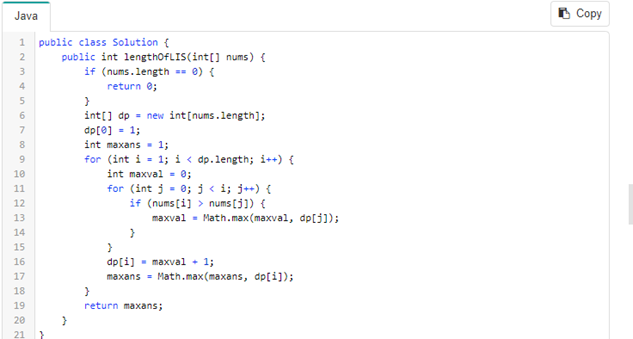### 一

``````第一層迴圈 ， 就是 走訪數列

4 會先跟 2 比大小 ，4比較大 ， 所以
Math.nax(4當前LIS , 2的LIS ) 。 決定要不要更新4 的LIS 。

maxans 最後就會是 dp[]裡最大的那個數字 。 (最大數字可能有很多一樣的，因為Longest Increasing Subsequence 可能有很多個)
``````

### 二

``````if (nums[i] > nums[j]) {
maxval = Math.max(maxval, dp[j]);
}

if (nums[i] >= nums[j]) {
maxval = Math.max(maxval, dp[j]);
}

``````

### 時間複雜度 :

time -- > 因為1+2+3+….n-1 ，會有 n * n 出現 (兩層迴圈)
space -- > 因為要多準備一個陣列 n size# 2 Dynamic Programming with Binary Search

``````input: [0, 8, 4, 12, 2]
dp: 
dp: [0, 8]
dp: [0, 4]
dp: [0, 4, 12]
dp: [0 , 2, 12]
``````
``````這個方法也是走訪每一個數字 ，

``````

### leetcode範例裡的解答看不懂 ，看這篇文章:

[LeetCode] 300. Longest Increasing Subsequence 最长递增子序列
https://www.cnblogs.com/grandyang/p/4938187.html

### 解法2 :

``````最小放dp ，最大放dp的最後面 。

``````

``````input: [0, 8, 4, 12, 2]

dp: 

dp: [0, 8]:

第一次
left =0 ，right = 2
mid = 0 +  2/2  = 1
if ( 8 < 4) left = mid+ 1
else right = mid       //( right = 1)

第二次
left =0 ，right = 1
mid = 0 +  1/2  = 0
if ( 0< 4) left = mid+ 1  // left = 1
else right = mid

之後
Ends [1 ]  = 4

``````

### 複雜度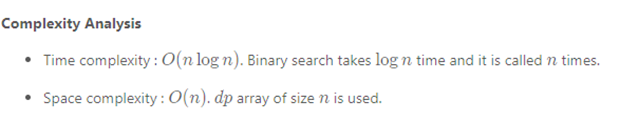``````因為 迴圈 長度 n ，每一輪Binary Search 都logn 。

``````

# 3 Brute Force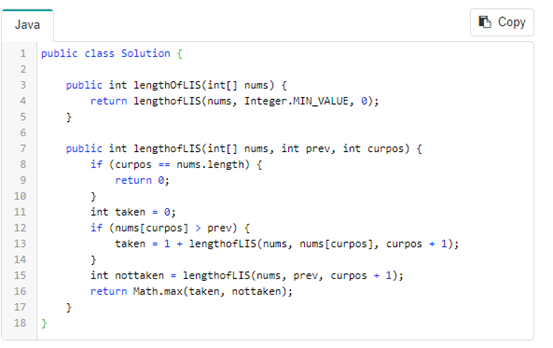``````Nums 是 數字陣列 。
Curpos  指的是current position ，現在在哪個陣列索引 。
Prev 指的是 上一個要比較的值(value) 。
``````

``````現在在2 ， 2 可以選擇 ，2可以不選擇 。

``````

## 複雜度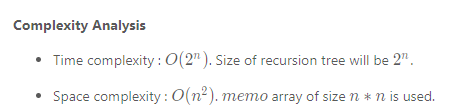### 時間複雜度

2.1.4 Recurrence Relation T(n)=2 T(n-1)+1 #4

# 4 Recursion with Memoization